# GMAT Math : Calculating the length of a side of a polygon

## Example Questions

### Example Question #1 : Calculating The Length Of A Side Of A Polygon

A pentagon with a perimeter of one mile has three congruent sides; one of the other sides is 100 feet longer than any of those three congruent sides, and the remaining side is 100 feet longer than that fourth side. What is the length of that longest side?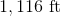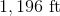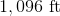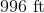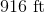Explanation:

If each of the five congruent sides has measure, then the other two sides have measures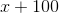and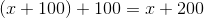. Add the sides to get the perimeter, which is equal to 5,280 feet, the solve for: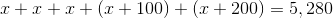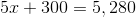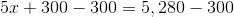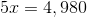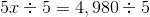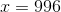Each of the shortest sides is 996 feet long; the longest side is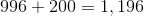feet long.

### Example Question #2 : Calculating The Length Of A Side Of A Polygon

The perimeter of a regular hexagon is one-half of a mile. Give the sidelength in inches.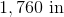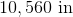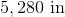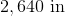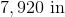Explanation:

One mile is 5,280 feet. The perimeter of the hexagon is one-half of this, or 2,640 feet. Since each side of a regular hexagon is congruent, the length of one side is one-sixth of this, or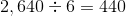feet.

Multiply by 12 to convert to inches: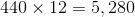inches.

### Example Question #3 : Calculating The Length Of A Side Of A Polygon

The perimeter of a regular pentagon is one-fifth of a mile. Give its sidelength in feet.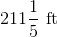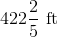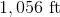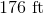Explanation:

One mile is 5,280 feet. The perimeter of the pentagon is one-fifth of this, or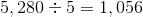feet. Since each side of a regular pentagon is congruent, the length of one side is one fifth-of this, or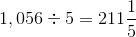feet.

### Example Question #1 : Calculating The Length Of A Side Of A Polygon

The perimeter of a regular octagon is two kilometers. Give its sidelength in meters.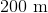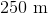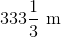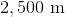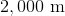Explanation:

One kilometer is equal to 1,000 meters, so two kilometers comprise 2,000 meters. A regular octagon has eight sides of equal length, so divide by 8 to get the sidelength: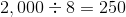meters.

### Example Question #2 : Calculating The Length Of A Side Of A Polygon

The perimeter of a regular pentagon is three kilometers. Give its sidelength in meters.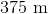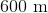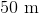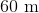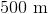Explanation:

One kilometer is equal to 1,000 meters, so two kilometers comprise 3,000 meters. A regular pentagon has five sides of equal length, so divide by 5 to get the sidelength: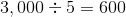meters.

### Example Question #6 : Calculating The Length Of A Side Of A Polygon

Six regular polygons each have perimeter one mile. The polygons are an equilateral triangle, a square, a pentagon, a hexagon, an octagon, and a decagon (ten-sided polygon). What is the mean of their sidelengths?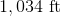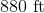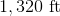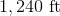Explanation:

The sidelength of a regular polygon is the perimeter of one mile, or 5,280 feet, divided by the number of sides. Therefore, the sidelengths of the six shapes are:

Triangle: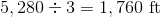Square: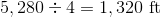Pentagon: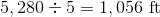Hexagon: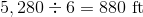Octagon: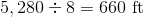Decagon: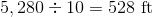The mean of the six sidelengths: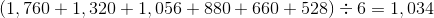feet

### Example Question #7 : Calculating The Length Of A Side Of A Polygon

If the shape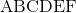is a regular hexagon with side length of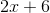and a perimeter ofmeters, what is the length of one side?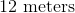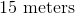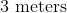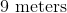Explanation:

Perimeter is the total distance around a shape. Here we have a regular (all sides are equal) hexagon with side distance 2x+6. Multiplying this expression by 6 gives us our perimeter.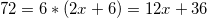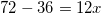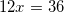But we are not done yet. We need to plug this back in to get the side length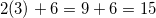so 15 meters

Alternatively, we could divide 72 by 6 to get 12 meters.

### Example Question #3 : Calculating The Length Of A Side Of A Polygon

A regular hexagon has perimeter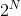. Which of the following is equal to the length of one side?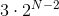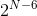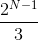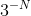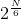Explanation:

A hexagon has six sides of equal length, so divide the perimeter by 6, which is the product of 2 and 3: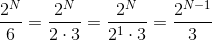### Example Question #4 : Calculating The Length Of A Side Of A Polygon

A regular octagon has perimeter. Give the length of one of its sides.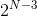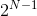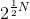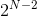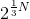Explanation:

A regular octagon has eight sides of equal length, so divide the perimeter by 8, or equivalently,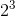: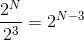.

Tired of practice problems?

Try live online GMAT Math prep today.